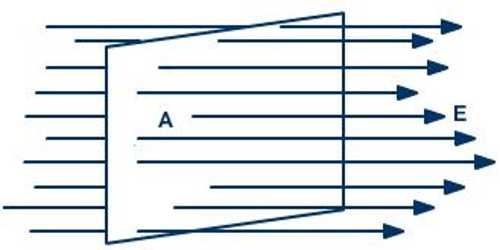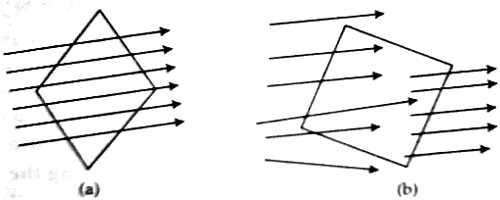Physics

# Electric FluxElectric flux: The number of electric lines of force or field lines passing through a plane or surface is called electric flux. It is denoted by φE.

(i) When the direction of electric field and the normal to the plane are parallel to each other, then electric flux is maximum [figure (a)].(ii) When the direction of electric field and the normal to the plane is perpendicular to each other, then electric flux is zero [figure (b)].

The electric flux through an area is defined as the electric field multiplied by the area of the surface projected in a plane perpendicular to the field. Electric field lines are considered to originate on positive electric charges and to terminate on negative charges. In order to determine the electric field intensity at a point due to more than one point charges, it is needed to determine the electric field intensity at that point for each individual point charge separately and the resultant field intensity will be the vector sum of all the individual field intensity. Now, if there is N number of point charges, then the resultant electric field intensity is,

E = E1 + E2 + E3 + …. …. + En = ΣEn

“The electric field intensity at a point in an electric field is 10 S. I. unit.” This statement means that at that point of the electric field 10 Newton force will act on 1 coulomb of positive charge.